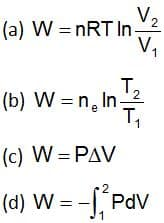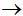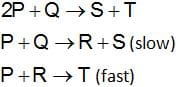# General Chemistry Questions and Answers### Basic Chemistry Questions with Answers:

Question: The chemical name of the bleaching powder is:
(a) Calcium oxide
(b) Sodium hydrogen carbonate
(c) Sodium bicarbonate
(d) Calcium oxychloride

Ans. (d)

Question: Which type of glass is used for making glass reinforced plastic?
(a) Fiber glass
(b) Quartz glass
(c) Flint glass
(d) Pyrex glass

Ans. (a)

Question: Which Charcoal adsorbs coloring matter and odoriferous gases ?
(a) Activated Charcoal
(b) Animal Charcoal
(c) Wood Charcoal
(d) All of these

Ans. (b)

Question: Sulfuric acid is also known as
(a) Oil of Vitriol
(b) Blue Vitriol
(c) Water Vitriol
(d) Acid Vitriol

Ans. (a)

Question: Which of the following are isomers?
(a) Ethane and Propane
(b) Methane & Met bene
(c) Propane & Propene
(d) Butane and Isobutane

Ans. (d)

Question: The permanent hardness of water is due to the presence of
(a) Calcium
(b) Sulfate
(c) Magnesium
(d) All of these

Ans. (d)

Question: Sulfur is not present in
(a) iron pyrites
(b) gypsum
(c) coal
(d) chlorapatite

Ans. (d)

Question: Which gas is used in atomic research and radiotherapy?
(a) Neon
(c) Propane
(d) Xenon

Ans. (d)

Question: The most important ore of Aluminum is —
(a) Bauxite
(b) Calamine
(c) Calcite
(d) Galena

Ans. (a)

Question: Which expression is correct for the work done on adiabatic reversible expansion of an ideal gasAns. (b)

Question: The element present in the largest number of rocks and minerals is —
(a) Carbon
(b) Silicon
(c) Hydrogen
(d) Aluminum

Ans. (b)

Question: An alloy used in making heating elements for electric heating devices is —
(a) Solder
(b) Alloysteel
(c) Nichrome
(d) German Silver

Ans. (c)

Question: __ is also known as Marsh gas
(a) Ethylene
(b) Acetylene
(c) Ethane
(d) Methane

Ans. (d)

Question: How does common salt help in separating soap from the solution after saponification?
(a) By decreasing the density of soap
(b) By decreasing solubility of Soap
(c) By increasing the density of soap
(d) By increasing the solubility of soap

Ans. (b)

Question: Which  of the following  is NOT a chemical change?
(a) Conversion of carbohydrate to energy
(b) Converting water into steam
(c) Making curd from milk
(d) Heating coal

Ans. (b)

Question: For reaction 2A + Bproducts, the active mass of B is kept constant and that of A is doubled. The rate of reaction will then
(a) Increase 2 times
(b) Increase 4 times
(c) Decrease 2 times
(d) Decrease 4 times

Ans. (b)

Question: German Silver is an alloy of —
(a) Copper, Silver & Nickel
(b) Silver, Copper & Aluminum
(c) Zinc, Copper & Nickel
(d) Silver, Zinc & Nickel

Ans. (c)

Related: Capital of Croatia and Currency

Question: Air is a/an —
(a) Compound
(b) Element
(c) Mixture
(d) Electrolyte

Ans. (c)

Question: Elements contain only __ type of atoms
(a) Single
(b) Many
(c) Same
(d) Different

Ans. (a)

Question: The substance that loses electrons is called:
(a) oxidizing agent
(b) reducing agent
(c) catalyst
(d) None of these

Ans. (b)

Question: Which of the following is the best conductor of electricity ?
(a) Ordinary water
(b) Sea water
(c) Boiled water
(d) Distilled water

Ans. (b)

Question: PbO2 is
(a) Basic
(b) Acidic
(c) Neutral
(d) Amphoteric

Ans. (d)

Question: Ionization energy is the energy required to remove an ___ from the outermost shell of an isolated gaseous atom
(a) Both electrons and protons
(b) Nuetron
(c) Proton
(d) Electron

Ans. (d)

Related: Clock test questions

Question: What is the effect of mixing manganese dioixide with hydrogen peroxide?
(a) The manganese dioxide breaks down into manganese and oxygen
(b) The hydrogen peroxide’s bleaching action is accelerated and increased
(c) Nothing
(d) The hydrogen peroxide breaks down into water and oxygen

Ans. (d)

Question: The element common to all acids is
(a) hydrogen
(b) carbon
(c) sulfur
(d) oxygen

Ans. (a)

Question: In an organic compound, which elements are generally present in addition to hydrogen?
(a) Phosphorus
(b) Sulfur
(c) Nitrogen
(d) Carbon

Ans. (d)

Question: Balloons are filled with —
(a) Helium
(b) Oxygen
(c) Nitrogen
(d) Argon

Ans. (a)

Question: In the Fischer-Tropsch synthesis of petrol….. and ….. are used as the raw materials
(a) H2 ; CO
(b) CH4 ; H2
(c) CH4 ; CH3OH
(d) CH3OH ; CO

Ans. (a)

Question: The charcoal used to decolorize raw sugar is —
(a) Animal charcoal
(b) Sugar charcoal
(c) Cocoanut charcoal
(d) Wood charcoal

Ans. (d)

Question: What is gypsum?
(a) Lime
(b) Bleaching powder
(c) Blue vitriol
(d) Ammonium chloride powder

Ans. (c)

Question: What is the name of the transition from a liquid state directly to a gas of an element or compound?
(a) Ionization
(b) Condensation
(c) Deionization
(d) Vaporization

Ans. (d)

Question: pH of lemon juice is 4. That means it is
(a) Basic
(b) Acidic
(c) Neutral
(d) None of these

Ans. (b)

Question: The gas used to extinguish a fire is —
(a) Neon
(b) Nitrogen
(c) Carbon dioxide
(d) Carbon Monoxide

Ans. (c)

Question: Arrange NH4+, H­2O, H3O+, HF and OHin increasing order of acidic nature
(a) H3O+ < NH4+ < HF < OH < H2O
(b) NH4+ < HF < H3O+ < H2O < OH
(c) OH < H2O < NH4+ < HF < H3O+
(d) H3O+ > HF > H2O > NH4+ > OH

Ans. (c)

Question: Drying oils contains a large proportion of
(a) Cholesterol
(b) Unsaturated fatty acids
(c) Fats
(d) Protein

Ans. (b)

Related: vector force problems

Question: In which of the following activities Silicon Carbide is used ?
(a) Making cement and glass
(b) Disinfecting water and ponds
(c) Making castes for statues
(d) Cutting very hard substances

Ans. (d)

Question: Popular use of which of the following fertilizers increases the acidity of soil?
(a) Ammonium Sulfate
(b) Potassium Nitrate
(c) Urea
(d) Superphosphate of lime

Ans. (a)

Question: The element common to all acids is —
(a) Oxygen
(b) Hydrogen
(c) Nitrogen
(d) Sulfur

Ans. (b)

Question: To make steel hard we add
(a) Silicon
(b) Carbon
(c) Chromium
(d) Manganese

Ans. (b)

Question: What is the chemical symbol for Manganese?
(a) Mo
(b) Mg
(c) Me
(d) Mn

Ans. (d)

Question: Marsh gas is
(a) nitrogen
(b) carbon
(c) methane
(d) hydrogen

Ans. (c)

Question: Gobar gas contains mainly —
(a) Methane
(b) Carbon dioxide
(c) Butane
(d) Carbon Monoxide

Ans. (a)

Question: Tetraethyl lead is used as —
(a) Mosquito repellent
(b) Pain Killer
(c) Fire extinguisher

Ans. (d)

Question: A white substance having an alkaline nature in solution is
(a) NaNO3
(b) NH4Cl
(c) Na2CO3
(d) Fe2O3

Ans. (c)

Question: What is the name for the temperature at which, theoretically, all molecular activity ceases and chemical reactions become impossible?
(a) Ground zero
(b) Freezing point
(c) Absolute zero
(d) Critical mass

Ans. (c)

Related: questions on Compound Interest

Question: Which of the following is used in beauty parlors for hair setting ?
(a) Phosphorus
(b) Sulfur
(c) Chlorine
(d) Silicon

Ans. (b)

Question: The chemical name of laughing gas is –
(a) Dinitrogen tetroxide
(b) Methane
(c) Nitrous oxide
(d) Ammonium Nitrate

Ans. (c)

Question: Tritium is an isotope of
(a) Oxygen
(b) Chlorine
(c) Hydrogen
(d) None of these

Ans. (c)

Question: Which of the following is not correct for N2O?
(a) It is called laughing gas
(b) It is nitrous oxide
(c) It is not a linear molecule
(d) It is least reactive in all oxides of nitrogen

Ans. (c)

Question: Quick Silver is the popular name of –
(a) Mercury
(b) Uranium
(c) Rubidium
(d) Sillicon

Ans. (a)

Related: Set theory questions

Question: Which of the following is the chief source of Napthalene?
(a) Moth ball
(b) Mothflakes
(c) Tar Camphor
(d) Coal tar

Ans. (d)

Question: A solution contains 2 components.Which is in less quantity is called as
(a) Substance
(b) Solvant
(c) Solution
(d) Solute

Ans. (d)

Question: Gay Lussac proposed which of the following ?
(a) Law of Gases
(b) Motion of Planets
(c) Theory of Elctromagnetism
(d) Law of motion

Ans. (a)

Question: The alkaline hydrolysis of oils or fats gives soap and:
(a) Glycerol
(b) Ethenol
(c) Glycol
(d) Ethanoic acid

Ans. (a)

Question: When sulfur is boiled with Na2SO3 solution, the compound formed is
(a) Sodium sulfide
(b) Sodium sulfate
(c) Sodium persulphate
(d) Sodium thiosulfate

Ans. (d)

Question: The reaction which converts sugar solution into alcohol is an example of
(a) saponification
(b) hydrogenation
(c) fermentation
(d) Hydrolysis

Ans. (c)

Question: Obsidian, caused naturally when lava cools very quickly, is what type of substance?
(a) Glass
(b) Pumice
(c) Crystal
(d) Granite

Ans. (a)

Question: When an iron nail gets rusted, iron oxide is formed.
(a) With reduction of weight
(b) Without any change in weight
(c) Without any change in color
(d) With increase in weight

Ans. (d)

Question: The yellow colour of Topaz crystal is due to the presence of
(a) Ferrous Oxide
(b) Nitrogen Oxide
(c) Calcium Oxide
(d) Aluminum fluorosilicate

Ans. (d)

Question: A saturated solution at S.T.P. is
(a) Colorless
(b) Green
(c) Red
(d) Red and green

Ans. (a)

Question: The volume of 1 g each of methane (CH4), ethane (C2H6), propane (C3H8) and butane (C4H10) was measured at  350  K  and  1 atm. What is  the  volume  of butane
(a) 495 cm3
(b) 600 cm3
(c) 900 cm3
(d) 1700 cm3

Ans. (a)

Question: The pure element in the following is
(a) Glass
(b) Diamond
(c) Lime
(d) Salt

Ans. (b)

Related: Space quiz questions

Question: Which of the following is not a chemical action?
(a) Burning of coal
(b) Conversion of water into steam
(c) Digestion of food
(d) Burning of paper

Ans. (b)

Question: The purest form of iron is
(a) Steel
(c) Stainless steel
(c) Cast iron
(d) Wrought iron

Ans. (d)

Question: Sub-atomic particles of atoms are __
(a) Only protons
(b) Only neutrons
(c) Both protons & neutrons
(d) Proton, electron & neutron

Ans. (d)

Question: The fundamental particles present in the nucleus of an atom are
(a) Electron, proton
(b) Proton, neutron
(c) Neutron, electron
(d) Neutron, positron

Ans. (b)

Question: Which of the following contains a coordinate covalent bond?
(a) N2H5+
(b) BaCl2
(c) HCl
(d) H2O

Ans. (a)

Question: Acids turn blue litmus into
(a) Green
(b) Red
(c)Yellow
(d) Orange

Ans. (b)

Related: Famous Places in the World quiz

Question: The state in which molecular attractions are very strong is .
(a) Solid
(b) Liquid
(c) Gas
(d) None of these

Ans. (a)

Question: Which is the most abundant element in the universe?
(a) Gold
(b) Oxygen
(c) Carbon
(d) Hydrogen

Ans. (d)

Question: The substance coated on plastic tape recorder tapes is
(a) Zinc oxide
(b) Magnesium oxide
(c) Iron sulfate
(d) Iron oxide

Ans. (d)

Question: Laws of electrolysis are given by:
(b) Maxwell
(c) Lenz
(d) Bohr

Ans. (a)

Question: Hemoglobin contains 0.33% of iron by weight. The molecular weight of hemoglobin is approximately 67200. The number of iron atoms (at wt. of Fe = 56) present in one molecule of hemoglobin are
(a) 1
(b) 2
(c) 4
(d) 6

Ans. (c)

Question: Formation is a 40% solution of:
(a) Methanol
(b) Methenal
(c) Methanoic acid
(d) None of these

Ans. (b)

Related: Ray optics quiz

Question: In which of the following oxidation shows a positive oxidation state?
(a) CO
(b) N2O
(c) NO
(d) F2O

Ans. (d)

Question: Atoms are electrically charged as
(a) Positive
(b) Negative
(c) Bi-positive
(d) Neutral

Ans. (d)

Question: Washing soda is the common name for
(a) Calcium carbonate
(b) Calcium bicarbonate
(c) Sodium carbonate
(d) Sodium bicarbonate

Ans. (c)

Question: Which acid is produced when milk gets sour?
(a) Tartaric acid
(b) Butyric acid
(c) Lactic acid
(d) Acetic acid

Ans. (c)

Question: A nitrogen hydrogen mixture initially in the moler of ratio of 1 : 3 reached equilibrium to form ammonia when 25% of the M2 had reacted. If the total pressure of the system was 21 atm, the partial pressure of ammonia at the equilibrium was
(a) 4.5 atm
(b) 3.0 atm
(c) 2.0 atm
(d) 1.5 atm

Ans. (b)

Question: Which of the following methods gives the average molecular weight of a polymer?
(a) sedimentation equilibrium method
(b) sedimentation velocity method
(c) light scattering method
(d) viscosity method

Ans. (b)

Question: Which of the following is not correctly matched ?
(b) Green vitriol : Copper sulfate
(c) Plaster of Paris : Calcium sulfate
(d) Calomel : Mercurous Chloride

Ans. (d)

Question: The law of multiple proportions was discovered by
(a) John Dalton
(b) Robert Brown
(c) Joseph Proust

Ans. (a)

Question: When KMnO4 is reduced with oxalic acid in an acidic medium, the oxidation number of Mn changes from
(a) 7 to 4
(b) 6 to 4
(c) 7 to 2
(d) 4 to 2

Ans. (c)

Question: Which of the following is not an element?
(a) Sodium
(b)Tin
(c) Water
(d) Carbon

Ans. (c)

Question: Molecules in Solids
(a) are free to move about
(b) cannot move
(c) interchange positions frequently
(d) are spherical in shape

Ans. (b)

Question: Water is used in hot water bottles because:
(a) it is easily obtained in pure form
(b) it has high specific heat
(c) it is cheaper and not harmful
(d) it is easy to heat water

Ans. (b)

Question: Among the following elements, which has the largest atomic size.
(a) Magnesium
(b) Phosphorus
(c) Lithium
(d) Sodium

Ans. (a)

Question: Which of the following diffuses at the fastest rate?
(a) Solid
(b) Gas
(c) Liquid
(d) None of these

Ans. (b)

Question: Which of the following processes of a colloidal suspension is used to determine the nature of charge on the particles?
(a) Dialysis
(b) Electrophoresis
(c) Sedimentation
(d) Ultrafiltration

Ans. (b)

Question: Which of the following has an equal number of neutrons and protons?
(a) Hydrogen
(b) Deuterium
(c) iodine
(d) Chlorine

Ans. (b)

Related: amino acids mcqs with answers

Question: pH is the concentration of ions of:
(a) Hydrogen
(b) Chloride
(c) Sodium
(d) Sulfate

Ans. (a)

Question: Which of the following is the heavy isotope of hydrogen?
(a) Gold
(b) Iron
(c) Thorium
(d) Deuterium

Ans. (d)

Question: Adhesive and cohesive forces are :
(a) Physical forces
(b) Chemical forces
(c) Electrical forces
(d) Magnetic  forces

Ans. (a)

Question: Which of the following is the product of phosphorylation?
(a) Phosphoglyceric acid
(b) Fructose-1, 6 diphosphate
(c) Diphosphoglyceric acid
(d) Pyruvic acid

Ans. (b)

Question: Gold numbers of x, y and z are 0.4, 0.002 and 10, respectively, then the correct order of their protective efficiency will be
(a) y > x > Z
(b) x > y > z
(c) z > y > x
(d) y > z > x

Ans. (a)

Question: When freshly precipitated Fe(OH)2 is boiled with water in the presence of a few drops of dilute HCl, then the hydrated ferric oxide sol is formed. This method is an example of
(a) Solubilization
(b) Ultramicrofiltration
(c) Peptization
(d) Tyndall effect

Ans. (c)

Related: kingdom protista mcqs test

Question: Given the electronegativities of three elements x = 1.0, y = 2.5, z = 3.0, the types of bonding formed between xz and yz respectively would be
(a) Covalent, ionic
(b) Ionic, covalent
(c) Polar covalent, covalent
(d) None of these

Ans. (b)

Question: Which of the following is not a appropriate test for aniline?
(a) Isocyanide test
(b) Dye test
(c) Hypochlorite test
(d) Baeyer permanganate test

Ans. (d)

Question: A red-coloured solution is obtained by adding a few drops of HCl to freshly precipitated ferric hydroxide.
This phenomenon is called
(a) Peptisation
(b) Dialysis
(c) Protection reaction
(d) None of the above

Ans. (a)

Question: Which of the following cannot be used for obtaining ethylamine from acetamide?
(a) LiAlH4
(b) NA + EtOH
(c) Br2 + KoH
(d) Ni/H2

Ans. (c)

Question: Which of the following should be obtained on the reaction of formaldehyde in the presence of concentrated aqueous caustic alkali solution at room temperature?
(a) Sodium formate and ethyl alcohol
(b) Dark brown sticky polymer
(c) Methanol and formate ion
(d) Potassium salt of formic acid and alcohol

Ans. (c)

Question: In a vessel containing SO3, SO2 and O2 at equilibrium, some helium gas is introduced so that the total pressure increases while temperature and volume remains constant. According to the chateller principle, the dissociation of SO3
(a) Increase
(b) Decrease
(c) Remain unaltered
(d) Changes unpredictable

Ans. (c)

Related: biology basic questions

Question: According to electrochemical series, the correct order of chemical reactivity with water is
(a) K > Zn > Cu > Mg
(b) K > Mg > Zn > Cu
(c) K > Mg > Cu > Zn
(d) Zn > K > Cu > Mg

Ans. (b)

Question: Which one of the following is inflammable ?
(a) Helium
(b) Nitrogen
(c) Oxygen
(d) Hydrogen

Question: The mechanism for the reaction is given belowThe rate law expression for the reaction is
(a) r = k [P]2[Q]
(b) r = k[P][Q]
(c) r = k[A][R]
(d) r = k[P]2

Ans. (b)

Question: Which of the following is/are inorganic gas(es)?
(a) Carbon monoxide
(b) Hydrogen sulfide
(c) Chlorine
(d) All of the above

Question: Which particle can be removed from a stable neutral atom with the least energy?
(a) An alpha particle
(b) An electron
(c) A neutron
(d) A proton

Ans. (b)

Question: When 3 gram glucose (Mol. wt. = 180) is dissolved in 60 gram water at 15°C, then the osmotic pressure of the solution will be
(a) 6.57
(b) 0.65
(c) 0.34
(d) 5.57

Ans. (a)

Question: Efficiency of a catalyst depends upon
(a) The size of its particle
(b) Its molecular weight
(c) Its solubility
(d) None of these

Ans. (a)

Question: Which of the following is an organic gas?
(a) Hydrocarbons
(b) Aldehydes
(c) Ketones
(d) Ammonia